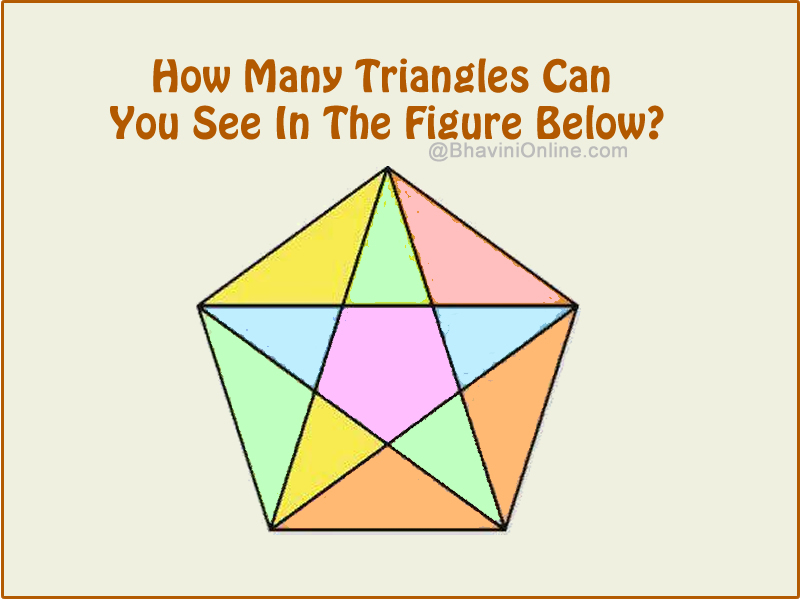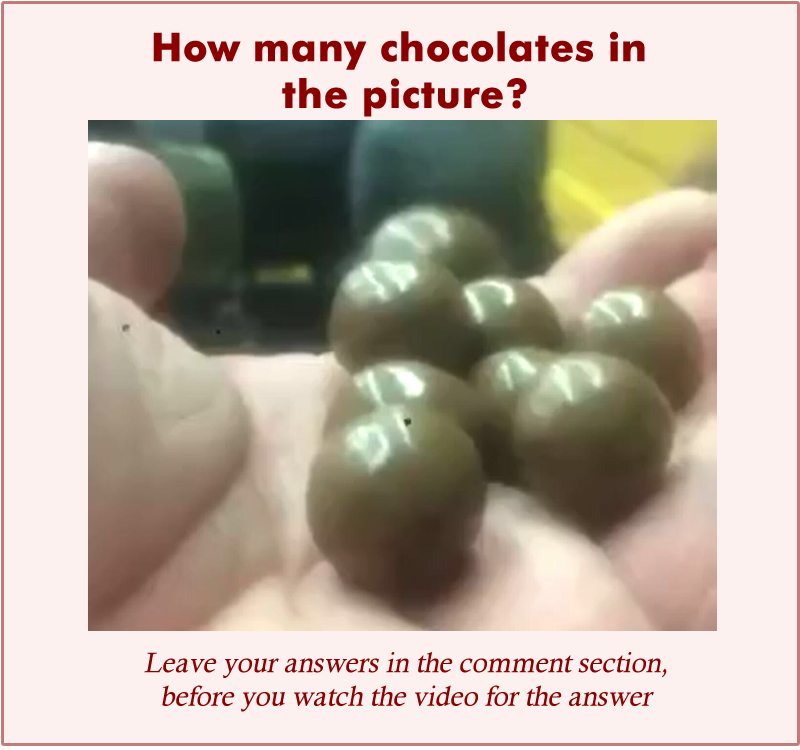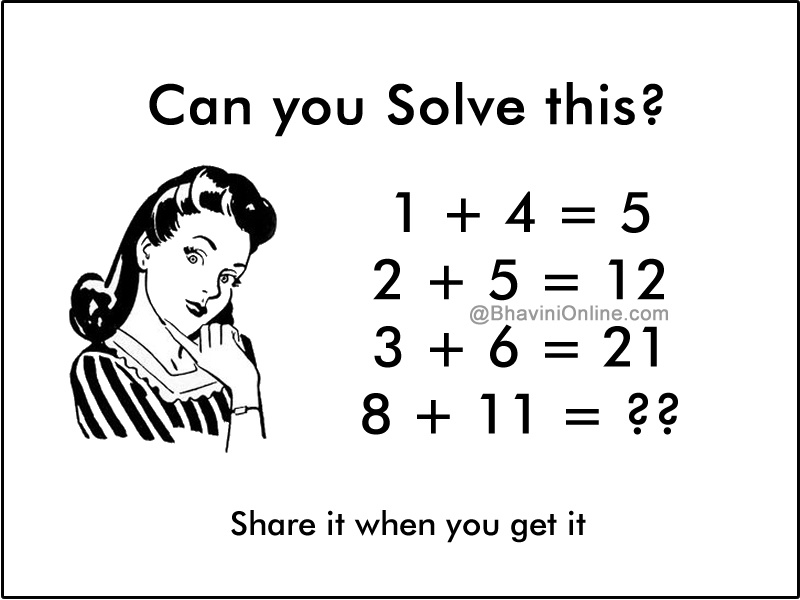# Top 10 Riddles and Puzzles of 2016 (1 to 5)

As promised I am back with the Top 10 list.

If you have missed the top 6 to 10 you can check them here.

Here is a list of Top 5 riddles and puzzles of 2016.

5. How Many Triangles Can You See In The Figure Below?

Count the number of triangles in the picture below;4. Fun Math Riddle: Find the Sum of Flowers

In the picture are given some equations from which you can find the value of the flowers and hence find the sum of all 3 flowers in the picture.3. Picture Riddle: How Many Chocolates in the Hand?

How many chocolates are there in the picture?2. Fun Maths Riddle: If 1 + 4 = 5; Then 8 + 11 = ??

If;

1 + 4 = 5

2 + 5 = 12

3 + 6 = 21

Then;

8 + 11 = ??And the No.1 Riddle of 2016 is…..

1. WhatsApp Riddle: Is it a Murder or Suicide?